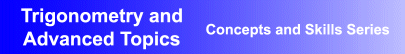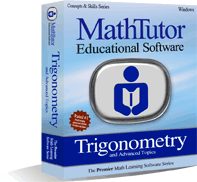Award-Winning

Trigonometry Interactive Learning SoftwareDESCRIPTION
TRIGONOMETRY AND ADVANCED TOPICS is the ideal

It is

trigonometry educational software

at its finest.
Parents seeking homeschool trigonometry instructional tutorial and learning software will find the program to be perfectly suited to their needs.
Educators seeking

curriculum-based trigonometry academic instructional learning software

will find the program to be ideal.
And individuals wanting interactive high school trigonometry computer tutorial lessons that will help them learn at their own pace will find the program to be exactly what they were looking for.
TRIGONOMETRY AND ADVANCED TOPICS has been acclaimed for its educational excellence as well as its flexibility. It can be used for self-study and to supplement classroom instruction in high school math classes.
Designed by math educators and in accordance with math curriculum standards, the program provides interactive trigonometry lessons suitable for students at all levels of ability.
Examples and trigonometry problem solving exercises progress gradually from the simpler to the more challenging, allowing students to build their skills and confidence.
• Award-winning, curriculum-based trigonometry courseware developed by math educators
• Provides dynamic, interactive trigonometry lessons that are ideal for self-study, and as a supplement to classroom instruction
• Demonstrated success in boosting students' math skills and comprehension, and proficiency in problem solving
• Network versions contain student record-keeping, grading, and performance analysis features
AVAILABLE FOR WINDOWS
• Windows XP/Vista
• Windows 7/8
• Windows 10
PRICING Single User Version: \$49.95TOPICS COVERED
Trigonometric Functions
• Definition of Angle
• Defining Trigonometric Functions
• Calculating Sine and Cosine
• Graphing Sine and Cosine
• Graphing Trigonometric Functions
• Trig. Functions of Angle Measures
Identities, Formulas, Equations
• Simplifying Trigonometric Expressions
• Sums and Differences
• Double Angle Formula
• Trigonometric Equations
Right Triangles
• Right Triangles I
• Right Triangles II
• Law of Cosines
• Law of Sines
Series and Progressions
• Arithmetic Progressions
• Arithmetic Series
• Geometric Progressions
• Geometric Series
• Binomial Theorem
Probability
• Permutations
• Combinations
• Probability
• Introductory Statistics